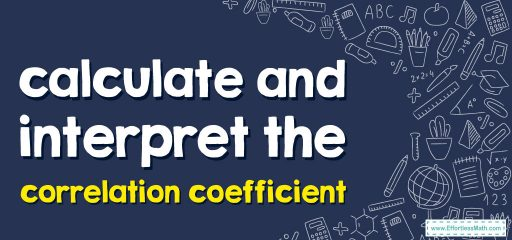# How to Calculate and Interpret Correlation CoefficientsA method of numerical measurement of the strength and direction of the relationship between two variables is to use the correlation coefficient.

There are different types of correlation coefficients, the most common of which are Pearson’s $$R$$-Rho and Spearman.

## Calculate Pearson’s R

The following formula is used to calculate Pearson’s $$R$$:

$$r=\frac{(nΣ(xy)-(Σx)(Σy))}{\sqrt{((nΣ(x^2)-(Σx)^2)(nΣ (y^2) – (Σy)^2))}}$$

Where:

• $$R$$ is the number of data points

• $$x$$ and $$y$$ are two variables

• $$Σx$$ and $$Σy$$ are the sum of $$x$$ and $$y$$ values, respectively

• $$Σxy$$ is the sum of the product of $$x$$ and $$y$$ values

• $$Σx^2$$ and $$Σy^2$$ are sums of squares of $$x$$ and $$y$$ values, respectively

## Calculate Spearman’s Rho

The following formula is used to calculate Spearman’s Rho:

rho$$=1-\frac{6∑d^2}{n^3-n}$$

where:

• $$n$$ is equal to the number of data points

• $$D$$ is the difference between the ranks of two variables ($$x$$ and $$y$$).

You can interpret the correlation coefficient using the following tips:

• When the correlation coefficient is $$+1$$, it is a complete positive linear relationship, that is, as the value of one variable increases, the value of the other variable also increases.

• When the correlation coefficient is $$-1$$, a negative linear relationship is complete; as the value of one variable increases, the value of the other variable decreases.

• A correlation coefficient of $$0$$ does not indicate a linear relationship between two variables.

• The correlation coefficient between $$0$$ and $$1$$ (excluding $$0$$) indicates a positive linear relationship between the variables, the higher the coefficient, the stronger the relationship. Also, the correlation coefficient between $$0$$ and $$-1$$ (excluding $$0$$) indicates a negative linear relationship between variables, a lower coefficient makes the relationship stronger.

### What people say about "How to Calculate and Interpret Correlation Coefficients - Effortless Math: We Help Students Learn to LOVE Mathematics"?

No one replied yet.

X
30% OFF

Limited time only!

Save Over 30%

SAVE $5 It was$16.99 now it is \$11.99